Courses

# Test: Venn Diagrams- 5

## 30 Questions MCQ Test Logical Reasoning (LR) and Data Interpretation (DI) | Test: Venn Diagrams- 5

Description
This mock test of Test: Venn Diagrams- 5 for SSC helps you for every SSC entrance exam. This contains 30 Multiple Choice Questions for SSC Test: Venn Diagrams- 5 (mcq) to study with solutions a complete question bank. The solved questions answers in this Test: Venn Diagrams- 5 quiz give you a good mix of easy questions and tough questions. SSC students definitely take this Test: Venn Diagrams- 5 exercise for a better result in the exam. You can find other Test: Venn Diagrams- 5 extra questions, long questions & short questions for SSC on EduRev as well by searching above.
QUESTION: 1

### In the following diagram the boys who are athletic and are disciplined are indicated by which number?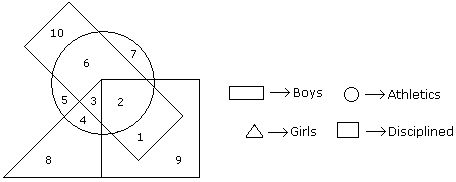Solution:

The required number is 2 because its common in the three shapes.

QUESTION: 2

### The diagram given below represents those students who play Cricket, Football and Kabaddi.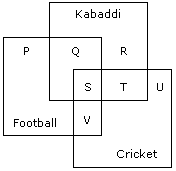Study the diagram and identify the students who play all the three games.

Solution:

S indicates those students who play all three games.

QUESTION: 3

### In an organization of pollution control board, engineers are represented by a circle, legal experts by a square and environmentalist by a triangle. Who is most represented in the board as shown in the following figure ?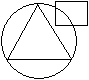Solution:

Environmentalists with Engineering background is most represented in the board.

QUESTION: 4

In the following figure triangle represents 'girls', square players and circle-coach. Which part of the diagram represents the girls who are player but not coach?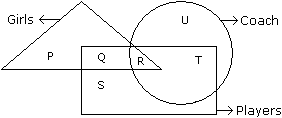Solution:

Q part of the figure represents those girls who are players but not coach.

QUESTION: 5

In the figure given below, square represents doctors, triangle represents ladies and circle represents surgeon. By which letter the ladies who doctor and surgeon both are represented ?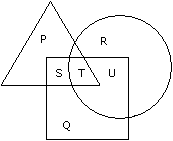Solution:
QUESTION: 6

Study the diagram and identify the people who can speak only one language.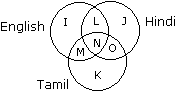Solution:

The regions represented by the letters K, J and I denote such people who can speak only one language.

QUESTION: 7

In the given figure if Triangle represents healthy people, Square represents old persons and Circle represents men then What is the number of those men who are healthy but not old ?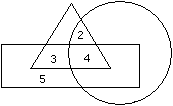Solution:
QUESTION: 8

Directions to Solve

In the following figure small square represents the persons who know English, triangle to those who know Marathi, big square to those who know Telugu and circle to those who know Hindi. In the different regions of the figures from 1 to 12 are given.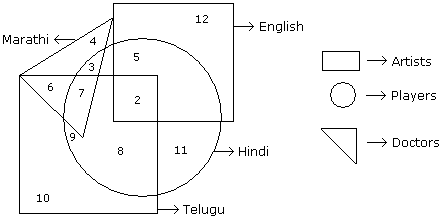Question -

How many persons can speak English and Hindi both the languages only ?

Solution:

Number of persons who can speak English and Hindi both only is 5.

QUESTION: 9

Directions to Solve

In the following figure small square represents the persons who know English, triangle to those who know Marathi, big square to those who know Telugu and circle to those who know Hindi. In the different regions of the figures from 1 to 12 are given.Question -

How many persons can speak Marathi and Telugu both ?

Solution:
QUESTION: 10

Directions to Solve

In the following figure small square represents the persons who know English, triangle to those who know Marathi, big square to those who know Telugu and circle to those who know Hindi. In the different regions of the figures from 1 to 12 are given.Question -

How many persons can speak English, Hindi and Telugu ?

Solution:

Number of persons who can speak English, Hindi and Telugu is 2.

QUESTION: 11

Directions to Solve

In the following figure small square represents the persons who know English, triangle to those who know Marathi, big square to those who know Telugu and circle to those who know Hindi. In the different regions of the figures from 1 to 12 are given.Question -

How many persons can speak only English ?

Solution:

Number of persons were can speak English is 12.

QUESTION: 12

Directions to Solve

In the following figure small square represents the persons who know English, triangle to those who know Marathi, big square to those who know Telugu and circle to those who know Hindi. In the different regions of the figures from 1 to 12 are given.Question -

How many persons can speak all the languages ?

Solution:

There is no such person who can speak all the languages.

QUESTION: 13

Directions to Solve

Study the diagram given below and answer each of the following questions.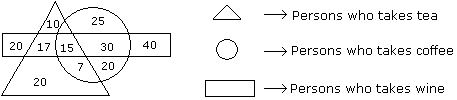Question -

How many persons who take tea and wine but not coffee ?

Solution:

17 persons take tea and wine but not coffee.

QUESTION: 14

Directions to Solve

Study the diagram given below and answer each of the following questions.Question -

How many persons are there who take both tea and coffee but not wine ?

Solution:

Number of persons was taken both tea and coffee but not wine is 7.

QUESTION: 15

Directions to Solve

Study the diagram given below and answer each of the following questions.Question -

How many persons are there who takes only coffee ?

Solution:

25 + 20 = 45

QUESTION: 16

Directions to Solve

Study the diagram given below and answer each of the following questions.Question -

How many persons take wine ?

Solution:

122 persons take wine.

QUESTION: 17

Directions to Solve

Study the diagram given below and answer each of the following questions.Question -

How many persons takes all the three ?

Solution:

15 persons take all the three.

QUESTION: 18

Directions to Solve

Study the following figure and answer the questions given below.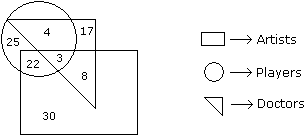Question -

How many doctors are neither artists nor players ?

Solution:

The number of doctors who are neither artists nor players is 17.

QUESTION: 19

Directions to Solve

Study the following figure and answer the questions given below.Question -

How many doctors are both players and artists ?

Solution:

The number of doctors who are both players and artists is 3.

QUESTION: 20

Directions to Solve

Study the following figure and answer the questions given below.Question -

How many artists are players ?

Solution:

The number of artists who are players is 22 + 3 = 25.

QUESTION: 21

Directions to Solve

Study the following figure and answer the questions given below.Question -

How many players are neither artists nor doctors ?

Solution:

The number of players who are neither artists nor doctors is 25.

QUESTION: 22

Directions to Solve

Study the following figure and answer the questions given below.Question -

How many artists are neither players nor doctors ?

Solution:

The number of artists who are neither players nor doctors is 30.

QUESTION: 23

Directions to Solve

In the following diagram rectangle represents men, Triangle represents educated, Circle represents urban and square represents government employees.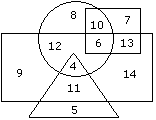Question -

Which one of the following represents the educated men but not urban ?

Solution:
QUESTION: 24

Directions to Solve

In the following diagram rectangle represents men, Triangle represents educated, Circle represents urban and square represents government employees.Question -

Which one of the following represents a woman who is urban as well as government employee ?

Solution:

Why women are not mentioned in the diagram?

Ans: Rectangle represents men, therefore the area outside the rectangle should be WOMEN.

How to find the answer for the this question?

Ans = [Woman & Urban & Government_Employee]

Conditions: Outside the rectangle(women) & Circle(urban) & Square(Govt. employee)

From the given diagram we can find that the value 10 is satisfies the above conditions.

QUESTION: 25

Directions to Solve

Study the following figure and answer the questions given below.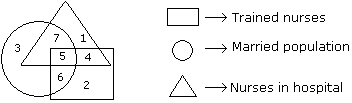Question -

If hospital management requires only married trained nurses for operation theater, which part of diagram should be chosen by him ?

Solution:

because the question is only married trained nurses, so the diagram circle, triangle (represents nurses in hospital) and rectangle where intersect is the married trained nurses to the hospital. So the answer is 5 only.

QUESTION: 26

Directions to Solve

Study the following figure and answer the questions given below.Question -

By which number, married but untrained nurses in the hospital are represented?

Solution:
QUESTION: 27

Directions to Solve

Study the following figure and answer the questions given below.Question -

By which numbers trained nurses are represented ?

Solution:

Option A = 3, 6 ; Here 3 is 'just only a married population'.
Option B = 7, 5 ; Here 7 is 'married nurses in hospital but not trained'.
Option D = 1, 1 ; Here 1 is 'nurses in hospital but not trained'.

But, Option C = 5, 6 ; Here both 5 & 6 are covered with 'Trained nurses'. Hence the required answers.

QUESTION: 28

Directions to Solve

Study the following figure and answer the questions given below.Question -

What is represented by the number 7 ?

Solution:
QUESTION: 29

Directions to Solve

Study the following figure and answer the questions given below.Question -

By which number, the trained unmarried nurses in the hospital are represented ?

Solution:

This is because in the given data states that there are only married, trained nurses in the hospital. But in the question it is asked as whether the nurse is unmarried. Eventually, the answer should be the left out number (the number in the triangle&square). So the answer is 4 only.

QUESTION: 30

Directions to Solve

Study the following figure and answer the questions given below.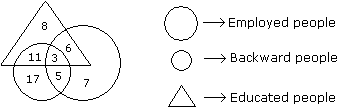Question -

How many educated people are employed ?

Solution:

Number of educated people who are employed = 3 + 6 = 9.